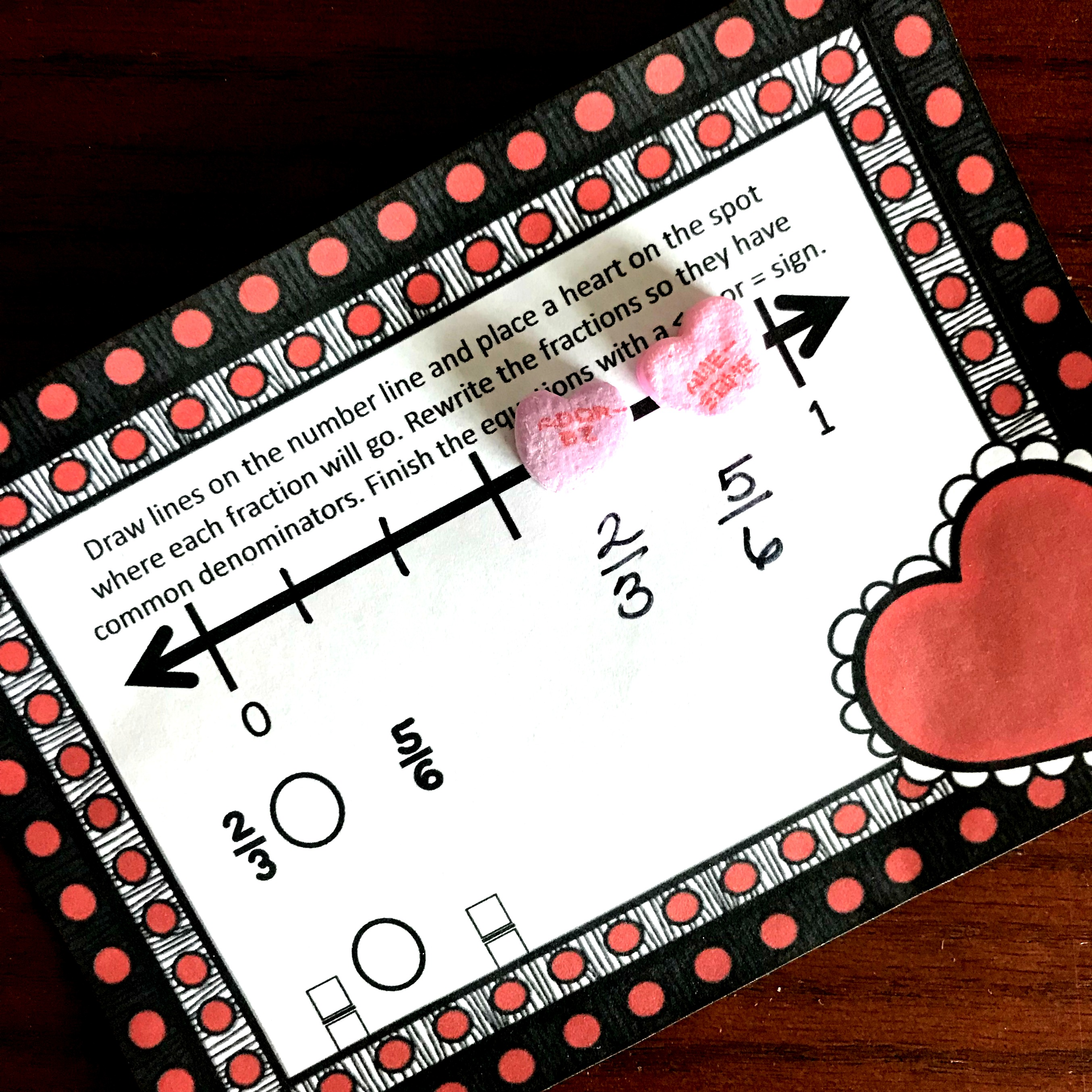# FREE Delicious Comparing Fractions on a Number Line Activity

Your children will love comparing fractions on a number line while using conversation hearts. It is a fun, hands-on way to work on ordering fractions!

It’s February, and that means hearts and candies are everywhere. Let’s embrace the fun of the season with this comparing fractions on a number line activity that uses conversational hearts as the manipulative.

## Prep-Work:

There are two different ways you can prep this.

Easy way (how I would do it):

Simply print the pages and place them in sheet protectors.

A little harder (but prettier):

1. First, print off the pages and cut out the task cards.
2. Next, laminate the task cards.

Then provide dry erase markers and conversational hearts, and you are ready to go.

## Comparing Fractions On a Number Line:

There are many different ways to compare fractions, but today we are going to look at two different strategies.

## Greater or less than 1/2?

One of the easiest ways to compare fractions is to determine if the fraction is larger or smaller than 1/2.

In this example, we have 3/4 and 1/3.

We know that half of 4 (the denominator) is 2. So if we have 3/4 then we have more than 1/2.

Next, we look at 1/3. Now we may not know what half of three is, but we can divide our number line into three equal groups. Now it is easy to see that 1/3 is less than 1/2. Therefore 1/3 < 3/4.

The first set of task cards focus on this skill right here. They can all be solved by finding which fraction is greater than 1/2 and less than 1/2.

## Finding the Common Denominator?

The next set has the children finding common denominators.

For example, they may get the card that asks to find out if 2/3 is greater than or less than 5/6.

To begin I skip count or list the multiples of 3 till I find one that six will go into too. In this case, that is very easy.

Three, six….oh six goes into six! Yea, I don’t have to change my 5/6 fractions.

Now, all I have to do is find the equivalent fraction of 2/3 which is 4/6. And when I place both of those fractions on the number line, it is easy to see that 2/3 is less than 5/6.

fractions-and-number-lines

You may also like:

Comparing-Fractions-on-A-Number-Line

Comparing fractions on a number line using clothespins:

Or get comparing fractions activities in this bundle.